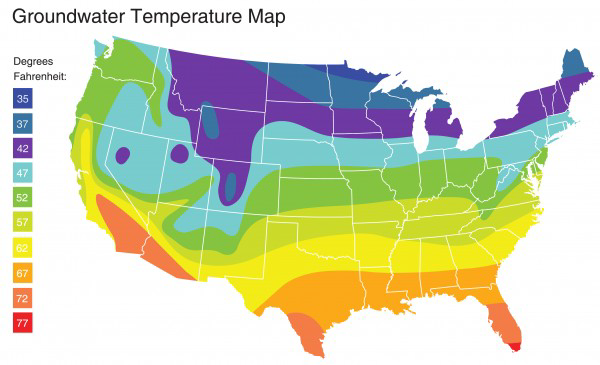# Calculating Required Wattage for Inline Water Heaters

Sizing: To determine wattage required, use the following equation:
GPM X TEMP RISE (°F) X 0.147 = kW REQUIRED
LPM X TEMP RISE (°C) X .07 = kW REQUIRED

Where GPM is gallons per minute of flow desired, temperature rise is expressed in degrees F.
Example: 4 GPM x 90°F x 0.147 = 52.9 kW (Use 53 kW)

The Inline Aquastorm Series Cleaner requires a minimum final DI rinse supply of 4-6 gpm @ 140-150 F
(Can vary depending on options and application)Note: Actual facility water temperature measurements should be used. Map is to be used a reference guide showing water temperatures will fluctuate depending on seasonal condition.

M
Michael is the author of this solution article.

Did you find it helpful? Yes No

Send feedback